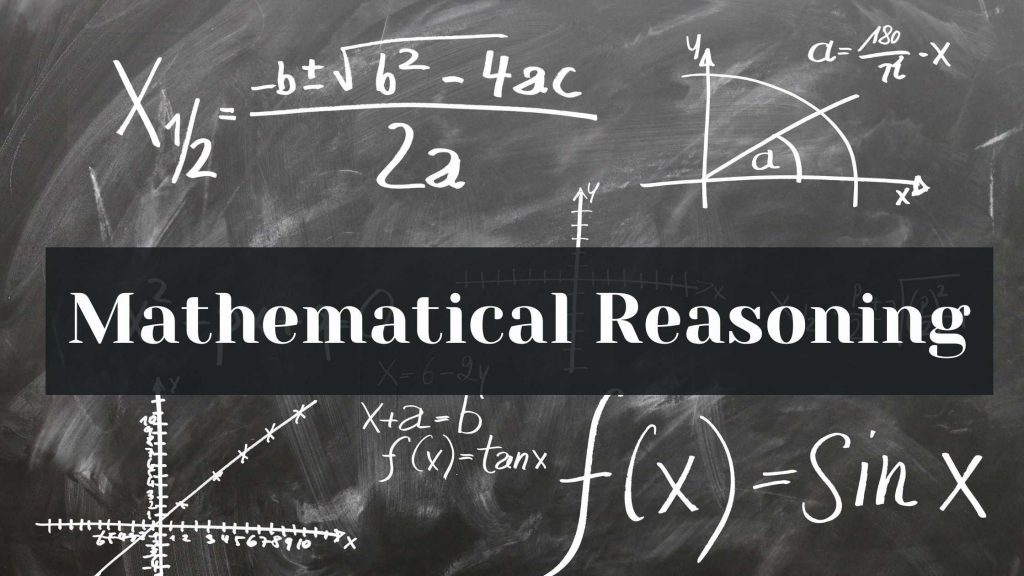Maths
Study Material

# Mathematical Reasoning using statements

Mathematical reasoning and the ability to communicate effectively are the two biggest boons of human kind to stay ahead of other species on this planet.

3 minutes long
Posted by Tanwir Silar, 28/7/2021Hesap Oluştur

Got stuck on homework? Get your step-by-step solutions from real tutors in minutes! 24/7. Unlimited.

We are going to discuss deductive reasoning in this blog. To learn about inductive reasoning refer to this blog.
Mathematical statements are the building blocks of reasoning. So, firstly let us learn about statements.(Mathematical Reasoning)

## What is a statement?

In mathematics, a sentence which is either false or true but not both is called a statement.
For Example:
1. Tigers are carnivores. ( T )
2. 8 is less than 6. ( F )
Ambiguous sentences are seldom considered as a statement.
For Example:
The sum of x and y is greater than 0.
Since, there is ambiguity about the trueness of the sentence, it can’t be regarded as a statement.

## Negation of a statement

The denial of a statement is called the negation of the statement. The negation of a statement p is denoted by ~p, which is also a statement.
For Example:
1. Let p: New Delhi is a city. The negation of the statement is New Delhi is not a city.
2. p: Every tiger is a mammal. The negation is Atleast a tiger is not a mammal.
For the last example, q: No tiger is a mammal cannot be considered as the negation of p. As the denial of particular statement doesn’t mean that every tiger is a mammal.
3. p: √7 is rational. The negation of this statement is √7 is not rational.
The statement q: √7 is irrational is not the negation because the denial of it does not equate to p.

## Compound Statements

The statement which is obtained by combining two or more statements using some connecting words like ‘and’, ‘or’, etc.,.
For Example:
1. Cricket is a team sport or an individual sport.
In the above statement, the two statements a) Cricket is a team sport b) Cricket is an individual sport. are combined using the connecting word ‘or’.

For Example:
2. Kohli plays football and hockey.
In the above example, the statements “Kohli plays football”, “Kohli plays hockey” are joined using the connecting word ‘and’.

## Connecting words “and” , “or”

AND
For a compound statement combined using ‘and’ to be true, all the component statements must be true.
If any of the component statement is false, then the compound statement is considered to be false.

OR
For a compound statement combined using ‘or’ to be false, all the component statements must be false, otherwise it is considered to be true.

## Implication or Implied statement

The statement of the form if p then q, is considered as an implication. It is also represented by p ⇒ q.
For Example:
r: If a number is a multiple of 9, then it is a multiple of 3.
The underlying statements are:
p: a number is a multiple of 9, q: a number is a multiple of 3.

## Contrapositive and Converse

For the implied statement, r: p ⇒ q , the statement t: ~q ⇒ ~p is called the contrapositive of r.
And the statement l: q ⇒ p is called the converse of r.
For Example:
r: If the physical environment changes, then the biological environment changes.
The contrapositive is t: If the biological environment does not change, then the physical environment does not change.
The converse is l: If the biological environment changes, then the physical environment changes.

We hope, our blog (mathematical reasoning) was helpful.